### Learn a new Times Table in only 5 days!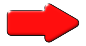Begin by writing out your times table on paper; slowly and carefully. Use addition to work out the next number in the sequence. Repeat this ten times then type out a neat copy to post onto the wall.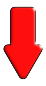### Fast Factors### Tables Conga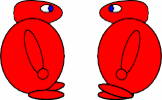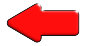### Tables Dash### Table Legs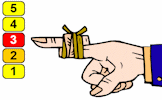### Tables Grab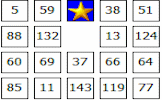Make up stories, jokes or rhymes to remind you of the difficult multiplication facts. Draw cartoon pictures to make them memorable. Post your work around the house or classroom.### Flash Tables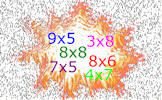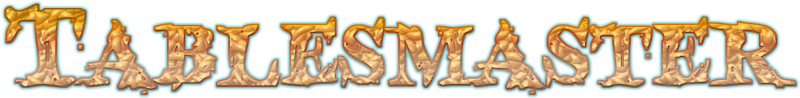The most popular activity on the Transum website!### Reverse Bar Chart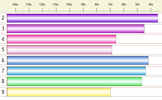### Beat The Clock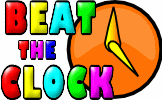### Playing Card Maths### Times Square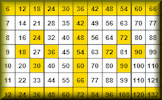### Expedite### Connect 4 Factors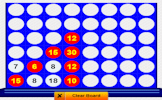### Grid Arithmetic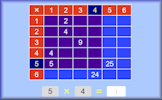### Hard   Times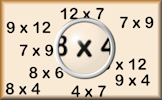Teachers: Start your lesson with a Times Tables Starter

Subscribers: Access our database of Times Tables Tips

### Times Tables

It is important to learn the times tables from 2 to 12. You should learn then as you'd learn a song or a dance. In fact you need to know your times tables forwards, backwards and all mixed up. Spend time learning them well and you'll reap the benefits in future.

Here are some printable forms on which you can record your progress: Personal Best Reverse Bar Chart | Tips For Remembering Multiplication Facts. Keep practising and one day you will be able to answer multiplication questions as fast as this:

The state of pupils' numeracy is always in the news and in particular politicians have pledged that every child in England will know their 12 times table before they leave primary school as part of a "war on innumeracy". They argue that there is still a case for learning your twelve times table, even though there are no longer 12 pence in a shilling, but the reason is to do with discovering patterns and building a confidence in handling numbers.

 1 x 1 = 12 x 1 = 23 x 1 = 34 x 1 = 45 x 1 = 56 x 1 = 67 x 1 = 78 x 1 = 89 x 1 = 910 x 1 = 1011 x 1 = 1112 x 1 = 12 1 x 2 = 22 x 2 = 43 x 2 = 64 x 2 = 85 x 2 = 106 x 2 = 127 x 2 = 148 x 2 = 169 x 2 = 1810 x 2 = 2011 x 2 = 2212 x 2 = 24 1 x 3 = 32 x 3 = 63 x 3 = 94 x 3 = 125 x 3 = 156 x 3 = 187 x 3 = 218 x 3 = 249 x 3 = 2710 x 3 = 3011 x 3 = 3312 x 3 = 36 1 x 4 = 42 x 4 = 83 x 4 = 124 x 4 = 165 x 4 = 206 x 4 = 247 x 4 = 288 x 4 = 329 x 4 = 3610 x 4 = 4011 x 4 = 4412 x 4 = 48 1 x 5 = 52 x 5 = 103 x 5 = 154 x 5 = 205 x 5 = 256 x 5 = 307 x 5 = 358 x 5 = 409 x 5 = 4510 x 5 = 5011 x 5 = 5512 x 5 = 60 1 x 6 = 62 x 6 = 123 x 6 = 184 x 6 = 245 x 6 = 306 x 6 = 367 x 6 = 428 x 6 = 489 x 6 = 5410 x 6 = 6011 x 6 = 6612 x 6 = 72 1 x 7 = 72 x 7 = 143 x 7 = 214 x 7 = 285 x 7 = 356 x 7 = 427 x 7 = 498 x 7 = 569 x 7 = 6310 x 7 = 7011 x 7 = 7712 x 7 = 84 1 x 8 = 82 x 8 = 163 x 8 = 244 x 8 = 325 x 8 = 406 x 8 = 487 x 8 = 568 x 8 = 649 x 8 = 7210 x 8 = 8011 x 8 = 8812 x 8 = 96 1 x 9 = 92 x 9 = 183 x 9 = 274 x 9 = 365 x 9 = 456 x 9 = 547 x 9 = 638 x 9 = 729 x 9 = 8110 x 9 = 9011 x 9 = 9912 x 9 = 108 1 x 10 = 102 x 10 = 203 x 10 = 304 x 10 = 405 x 10 = 506 x 10 = 607 x 10 = 708 x 10 = 809 x 10 = 9010 x 10 = 10011 x 10 = 11012 x 10 = 120 1 x 11 = 112 x 11 = 223 x 11 = 334 x 11 = 445 x 11 = 556 x 11 = 667 x 11 = 778 x 11 = 889 x 11 = 9910 x 11 = 11011 x 11 = 12112 x 11 = 132 1 x 12 = 122 x 12 = 243 x 12 = 364 x 12 = 485 x 12 = 606 x 12 = 727 x 12 = 848 x 12 = 969 x 12 = 10810 x 12 = 12011 x 12 = 13212 x 12 = 144Tablesmaster scores improve with a remote keypad

LogiLink Wireless Numeric Keypad### More Activities:

Do you have any comments? It is always useful to receive feedback and helps make this free resource even more useful for those learning Mathematics anywhere in the world. Click here to enter your comments.For All: